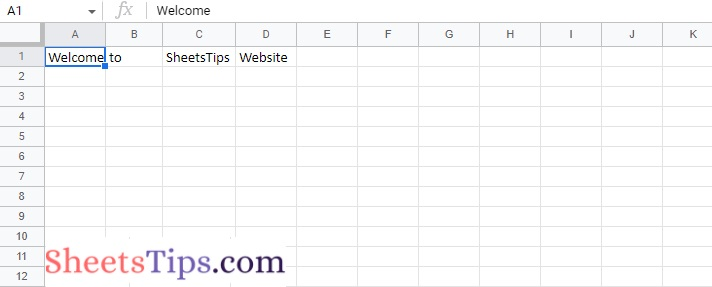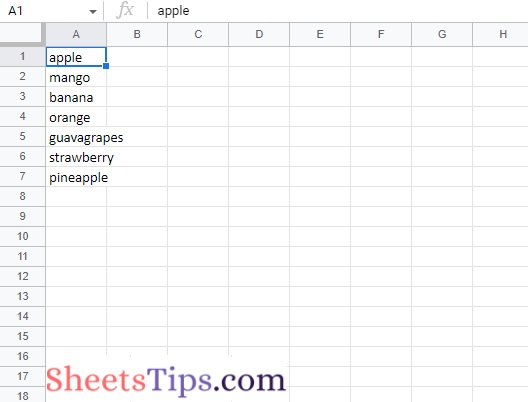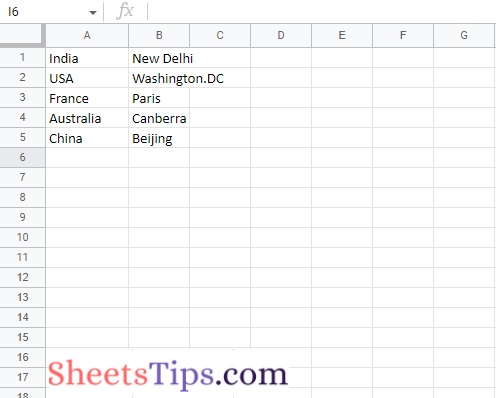# How to Create and Write on Excel File using xlsxwriter Module in Python

If you want to read or write data in an Excel file using Python, then you can simply use the XLSX writer module. Using the xlsxwriter function in Python, you can simply read or add information to the desired Excel file. Also, with the help of the xlsxwriter function, one can easily navigate through several excel worksheets and retrieve the data by specifying certain criteria. In this article, let’s understand everything XLSX writer and how to read and write an Excel file in Python using Pandas. Scroll down to find out more.

## How Do You Read and Write an Excel File in Python using Pandas? (XLSX Writer Module)

XlsxWriter is a Python module that allows you to write files in the XLSX file format. It can be used to create multiple spreadsheets with text, numbers, and formulas. It also allows formatting, images, charts, page setup, auto filters, conditional formatting, and many more capabilities.

Installation:

```pip install xlsxwriter
```

XlsxWriter Benefits and Drawbacks

XlsxWriter has certain benefits and drawbacks over other Python libraries for writing Excel files.

• It supports more Excel functionalities than the other modules.
• XlsxWriter offers a high degree of fidelity with Excel files. In most circumstances, the files produced are exactly the same as those produced by Excel.
• It comes with a lot of documentation, example files, and tests.
• XlsxWriter is fast and can be set to consume very less memory even when dealing with very big output files.

• XlsxWriter cannot read or modify existing Excel XLSX files.

Important Points to be Noted:

Rows and columns are zero-indexed throughout XlsxWriter. A1 is the first cell in a worksheet, i.e, A1 is (0, 0), B1 is (0, 1), A2 is (1, 0), B2 is (1, 1), and so on.
Let’s look at how to use Python to create and write an Excel spreadsheet.

## Python Code to Create and Write on Excel File using xlsxwriter

Below are the ways to create and write on Excel File using xlsxwriter:

### Method #1: Using A1 notation(cell name)

Here we are using A1 notation(cell name) to write data in the specific cells.

Approach:

• Import xlsxwriter module using the import keyword
• Pass the excel file path to the Workbook() function of xlsxwriter which is the file we want to create and store it in a variable
• Create the new Worksheet using the add_worsheet() function and apply it to the above workbook object and store it in a variable
• Apply write() function on the above new worksheet object to write the
data into the specific cells
• Close the workbook Object using the close() function.
• The Exit of the Program.

Below is the implementation:

```# Import xlsxwriter module using the import keyword
import xlsxwriter

# Pass the excel file path to the Workbook() function of xlsxwriter
# which is the file we want to create and store it in a variable
workbookObj = xlsxwriter.Workbook('demoExcelFile.xlsx')

# Create the new Worksheet using the add_worsheet() function and
# apply it to the above workbook object and store it in a variable

# Apply write() function on the above new worksheet object to write the
# data into the specific cells
newWorksheet.write('A1', 'Welcome')
newWorksheet.write('B1', 'to')
newWorksheet.write('C1', 'SheetsTips')
newWorksheet.write('D1', 'Website')

# Close the workbook Object using the close() function.
workbookObj.close()
```

Output:### Method #2: Using row-column notation(indexing value)

Here we are using row-column notation(indexing value) to write data in the specific cells.

Approach:

• Import xlsxwriter module using the import keyword
• Pass the excel file path to the Workbook() function of xlsxwriter which is the file we want to create and store it in a variable
• Create the new Worksheet using the add_worsheet() function and apply it to the above workbook object and store it in a variable
• Begin from the first cell.
• Take a variable and initialize the Row number and column number with 0(as they are zero-indexed)
and store them in two separate variables.
• Give some random list of data as static input and store it in another variable.
• Loop in the above data list using the for loop.
• Apply write() function on the above worksheet by passing row number, column number, and item as arguments to it to write the corresponding row and column cell with the item value
• Increment the row number by 1 after each iteration
• Close the workbook Object using the close() function.
• The Exit of the Program.

Below is the implementation:

```# Import xlsxwriter module using the import keyword
import xlsxwriter

# Pass the excel file path to the Workbook() function of xlsxwriter
# which is the file we want to create and store it in a variable
workbookObj = xlsxwriter.Workbook('SampleExcelFile.xlsx')

# Create the new Worksheet using the add_worsheet() function and
# apply it to the above workbook object and store it in a variable

# Begin from the first cell.
# Take a variable and initilaze the Row number and column number with 0(as they are zero-indexed)
# and store them in two separate variables.
rowNum= 0
colNum= 0

# Give some random list of data as static input and store it in another variable
data = ["apple", "mango", "banana", "orange", "guava"
"grapes", "strawberry", "pineapple", ]

# Loop in the above data list using the for loop
for each_item in data:

# Apply write() function on the above worksheet by passing row number, column number, item as
# arguments to it to write the corresponding row and column cell with the item value
newWorksheet.write(rowNum, colNum, each_item)

# Increment the row number by 1 after each iteration
rowNum += 1

# Close the workbook Object using the close() function.
workbookObj.close()
```

Output:### Method #3: Creating New Sheet

Here we create a new sheet with some random name.

Approach:

• Import xlsxwriter module using the import keyword.
• Pass the excel file path to the Workbook() function of xlsxwriter which is the file we want to create and store it in a variable.
• Create the new Worksheet using the add_worsheet() function by passing some random name to it and apply it to the above workbook object.
• It takes Sheet1, Sheet2, …. by default.
• Store it in a variable.
• Give some random data that we want to write it into the worksheet and store it as a tuple
• Begin from the first cell.
• Take two variables and initilaze the Row number and column number with 0(as they are zero-indexed).
• Loop in the above given data using the for loop and and write the data row by row into the above worksheet.
• Write the country data of the specified rowNumber and columnNumber cell with the country data value.
• Write the country data of the specified rowNumber and columnNumber cell +1 (next column) with the capital data value.
• Increment the row number by 1 after each iteration to insert the data in next row.
• Close the workbook Object using the close() function.
• The Exit of the Program

Below is the implementation:

```# Import xlsxwriter module using the import keyword
import xlsxwriter

# Pass the excel file path to the Workbook() function of xlsxwriter
# which is the file we want to create and store it in a variable
workbookObj = xlsxwriter.Workbook('new_worksheet.xlsx')

# Create the new Worksheet using the add_worsheet() function by passing
# some random name to it and apply it to the above workbook object.
# It takes Sheet1, Sheet2, .... by default.
# Store it in a variable.

# Give some random data that we want to write it into the worksheet and store it as a tuple
gvn_data = (
['India', 'New Delhi'],
['USA', 'Washington.DC'],
['France',  'Paris'],
['Australia', 'Canberra'],
['China', 'Beijing'],
)

# Begin from the first cell.
# Take two variables and initilaze the Row number and column number with 0(as they are zero-indexed)
rowNum= 0
colNum= 0

# Loop in the above given data using the for loop and and write the data row by row.
# into the above worksheet
for country, capital in (gvn_data):
# Write the country data of the specified rowNumber and columnNumber cell with the country data value
newWorksheet.write(rowNum, colNum, country)
# Write the country data of the specified rowNumber and columnNumber cell +1 (next column) with the capital data value
newWorksheet.write(rowNum, colNum + 1, capital)
# Increment the row number by 1 after each iteration to insert the data in next row
rowNum += 1

# Close the workbook Object using the close() function.
workbookObj.close()```

Output:Now that you have been provided with all the necessary information on how to read and write the Excel file in Python using the Pandas, With the simplest methods provided here, we can simply read and write an Excel file in Python just by using the Xlsxwriter libraries.